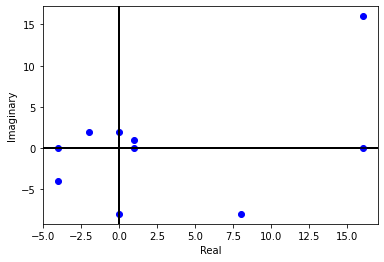# Argand Plane

A complex numbers $$x+iy$$ can be plotted in cartesian coordinate as the point $$(x,y)$$. This plane is called Argand plane.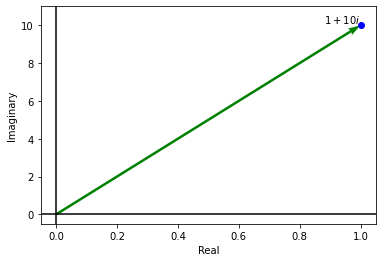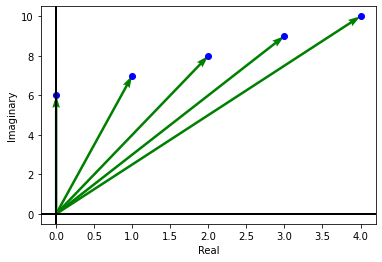Addition and substraction of complex numbers in Argand plane are similar to the vector addition and multiplication.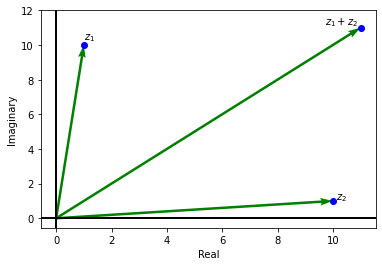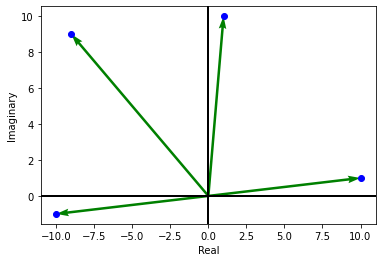## 1. Multiplication in Complex Numbers¶

If we multiply two complex number $$z_1=r_1e^{i\theta_1}$$ and $$z_2=r_2e^{i\theta_2}$$, then we get

$z_1\cdot z_2 = r_1\cdot r_2 e^{i (\theta_1 + \theta_2)}$

Hence in polar coordinate, the magnitude will get multiplies while angle will get added.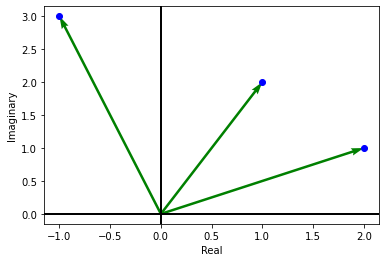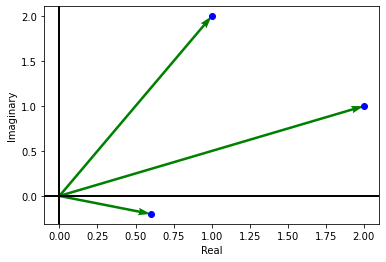## 2. Sequence and Series in Complex Numbers¶

Similar to real sequance, a complex sequence in simple a function $$f: \mathbb{N} \to \mathbb{C}$$.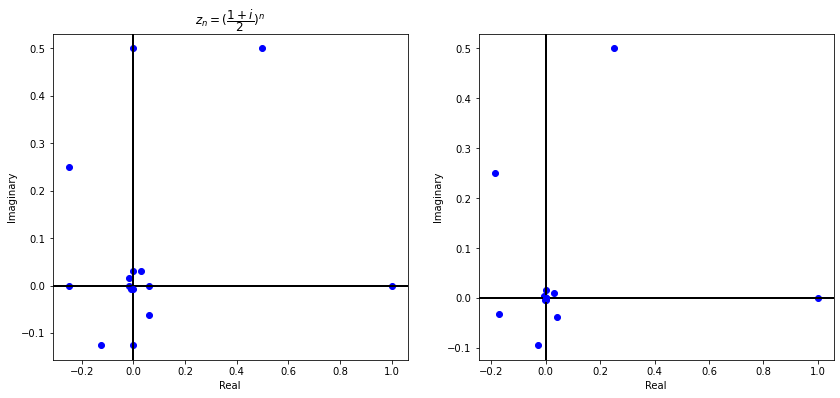Example of a diverging sequence.